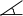# Level 4 - Math & Problem Solving

Our Level 4 Math & Problem Solving course targets students in Grades 8 to 10. This level addresses difficult topics in math and applies advanced problem-solving strategies.

Level 4 instruction includes, but is not limited to:

• Polynomials
• Linear equations and systems of linear equations
• Problem solving for linear equations, systems of equations, and for quadratic equations
• Geometry on a plane (axioms, theorems proof, problem solving)
• Introduction to trigonometry: trigo ratios, solving identities, sin and cosine laws, problem solving
• Arithmetic and geometric sets and series
• Mathematical induction
• Fundamental law of combinatorics, permutations, and combinations
• Logic problems – higher level content (see Levels 1 – 3)
• Brain teasers

Prerequisite for Level 4: Completion of Level 3 OR successful completion of assessment interview (for new students).

##### Sample problems

Given trapezoid ABCD. MN is a mid-line of ABCD. MN = 28cm.
Sides AB and CD continued until they meet in point P.APD = 30°.
A circle with diameter MN is drawn with the center O in
the middle of mid-line MN. Points B and C are on the circle.Stephanie and Elizabeth live in houses on the same street. The street is straight and there is a very high transmission antenna between their houses. Stephanie observes from her house the top of the antenna at the angle of elevation 33°, but Elizabeth observes from her house the top of the antenna at the angle of elevation 47°. How high is the top of the antenna from the ground, if Elizabeth’s house is 120 meters nearer to the antenna than Stephanie’s house?

Prove the following trigonometric identity:Prove the following using mathematical induction:
1 + 3 + 5 + 7 + . . . + (2n – 1) = n2